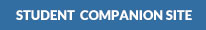Kimmel, Weygandt, Kieso:

Financial Accounting: Tools for Business Decision Making, 8th Edition•PowerPoint

•Excel Templates

•PowerPoint

•Excel Templates

•PowerPoint

•Excel Templates

•PowerPoint

•Excel Templates

•PowerPoint

•Excel Templates

•PowerPoint

•Excel Templates

•PowerPoint

•Excel Templates

•PowerPoint

•Excel Templates

•PowerPoint

•Excel Templates

•PowerPoint

•Excel Templates

•PowerPoint

•Excel Templates

•PowerPoint

•Excel Templates

•PowerPoint

•Excel Templates

## Get Help With:

* These links will open a new window